This book is archived and will be removed July 6, 2022. Please use the updated version.

Alternating-Current Circuits

# 102 RLC Series Circuits with AC

### Learning Objectives

By the end of the section, you will be able to:

• Describe how the current varies in a resistor, a capacitor, and an inductor while in series with an ac power source
• Use phasors to understand the phase angle of a resistor, capacitor, and inductor ac circuit and to understand what that phase angle means
• Calculate the impedance of a circuit

The ac circuit shown in (Figure), called an RLC series circuit, is a series combination of a resistor, capacitor, and inductor connected across an ac source. It produces an emf of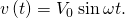(a) An RLC series circuit. (b) A comparison of the generator output voltage and the current. The value of the phase difference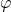depends on the values of R, C, and L.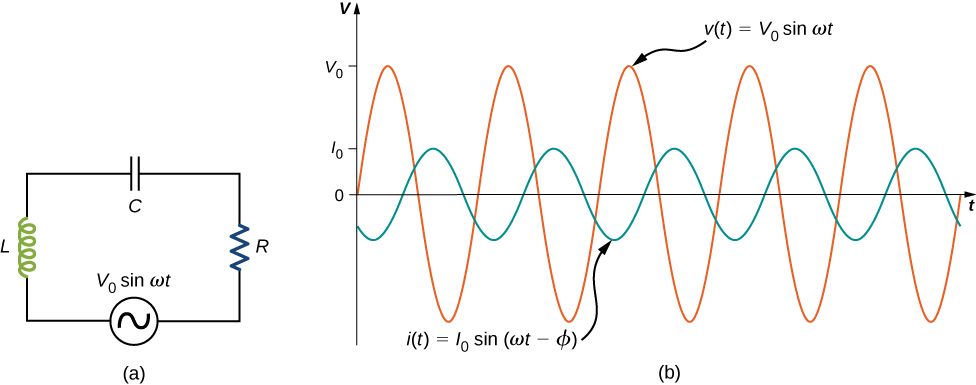Since the elements are in series, the same current flows through each element at all points in time. The relative phase between the current and the emf is not obvious when all three elements are present. Consequently, we represent the current by the general expression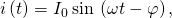where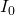is the current amplitude andis the phase angle between the current and the applied voltage. The phase angle is thus the amount by which the voltage and current are out of phase with each other in a circuit. Our task is to find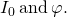A phasor diagram involving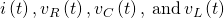is helpful for analyzing the circuit. As shown in (Figure), the phasor representing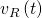points in the same direction as the phasor for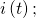its amplitude is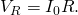The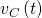phasor lags the i(t) phasor by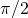rad and has the amplitude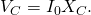The phasor for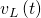leads the i(t) phasor byrad and has the amplitude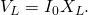The phasor diagram for the RLC series circuit of (Figure).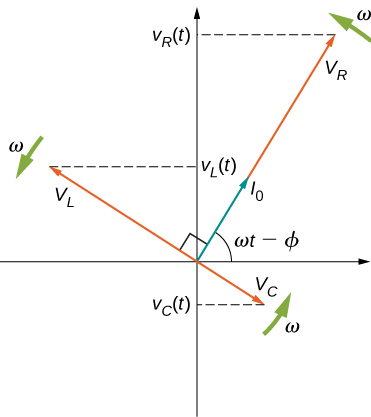At any instant, the voltage across the RLC combination is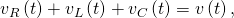the emf of the source. Since a component of a sum of vectors is the sum of the components of the individual vectors—for example,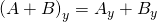—the projection of the vector sum of phasors onto the vertical axis is the sum of the vertical projections of the individual phasors. Hence, if we add vectorially the phasors representing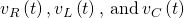and then find the projection of the resultant onto the vertical axis, we obtain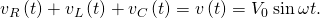The vector sum of the phasors is shown in (Figure). The resultant phasor has an amplitude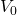and is directed at an anglewith respect to the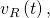or i(t), phasor. The projection of this resultant phasor onto the vertical axis isWe can easily determine the unknown quantitiesandfrom the geometry of the phasor diagram. For the phase angle,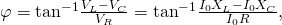and after cancellation of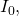this becomes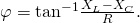Furthermore, from the Pythagorean theorem,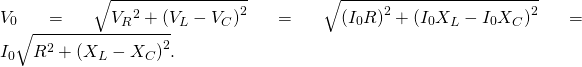The resultant of the phasors for,, andis equal to the phasor forThe i(t) phasor (not shown) is aligned with thephasor.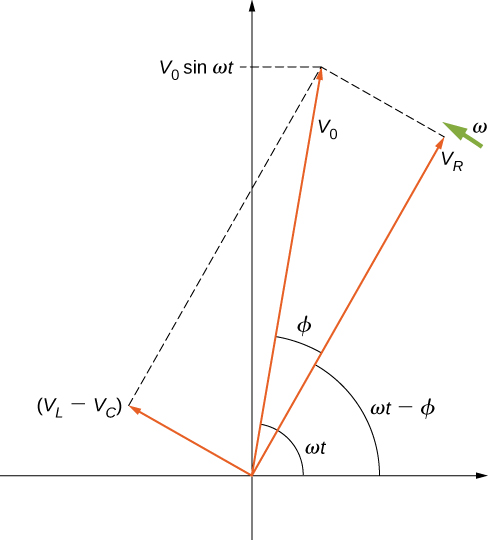The current amplitude is therefore the ac version of Ohm’s law: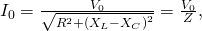where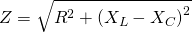is known as the impedance of the circuit. Its unit is the ohm, and it is the ac analog to resistance in a dc circuit, which measures the combined effect of resistance, capacitive reactance, and inductive reactance ((Figure)).

Power capacitors are used to balance the impedance of the effective inductance in transmission lines.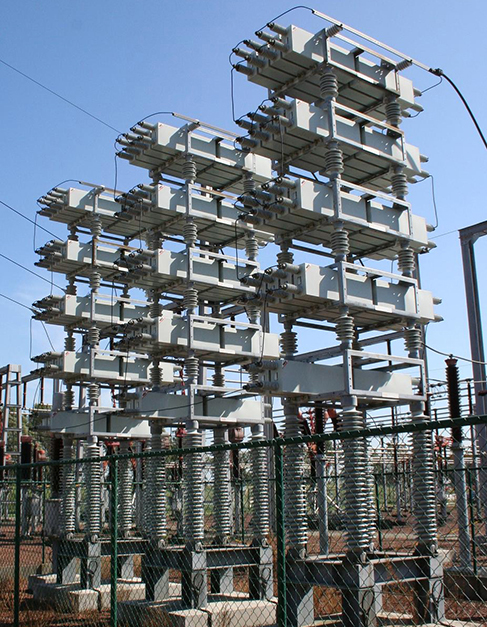The RLC circuit is analogous to the wheel of a car driven over a corrugated road ((Figure)). The regularly spaced bumps in the road drive the wheel up and down; in the same way, a voltage source increases and decreases. The shock absorber acts like the resistance of the RLC circuit, damping and limiting the amplitude of the oscillation. Energy within the wheel system goes back and forth between kinetic and potential energy stored in the car spring, analogous to the shift between a maximum current, with energy stored in an inductor, and no current, with energy stored in the electric field of a capacitor. The amplitude of the wheel’s motion is at a maximum if the bumps in the road are hit at the resonant frequency, which we describe in more detail in Resonance in an AC Circuit.

On a car, the shock absorber damps motion and dissipates energy. This is much like the resistance in an RLC circuit. The mass and spring determine the resonant frequency.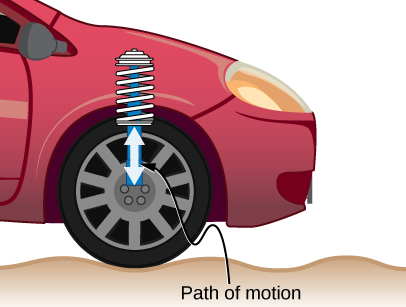Problem-Solving Strategy: AC Circuits

To analyze an ac circuit containing resistors, capacitors, and inductors, it is helpful to think of each device’s reactance and find the equivalent reactance using the rules we used for equivalent resistance in the past. Phasors are a great method to determine whether the emf of the circuit has positive or negative phase (namely, leads or lags other values). A mnemonic device of “ELI the ICE man” is sometimes used to remember that the emf (E) leads the current (I) in an inductor (L) and the current (I) leads the emf (E) in a capacitor (C).

Use the following steps to determine the emf of the circuit by phasors:

1. Draw the phasors for voltage across each device: resistor, capacitor, and inductor, including the phase angle in the circuit.
2. If there is both a capacitor and an inductor, find the net voltage from these two phasors, since they are antiparallel.
3. Find the equivalent phasor from the phasor in step 2 and the resistor’s phasor using trigonometry or components of the phasors. The equivalent phasor found is the emf of the circuit.

An RLC Series Circuit The output of an ac generator connected to an RLC series combination has a frequency of 200 Hz and an amplitude of 0.100 V. If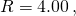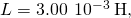and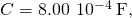what are (a) the capacitive reactance, (b) the inductive reactance, (c) the impedance, (d) the current amplitude, and (e) the phase difference between the current and the emf of the generator?

Strategy The reactances and impedance in (a)–(c) are found by substitutions into (Figure), (Figure), and (Figure), respectively. The current amplitude is calculated from the peak voltage and the impedance. The phase difference between the current and the emf is calculated by the inverse tangent of the difference between the reactances divided by the resistance.

Solution

1. From (Figure), the capacitive reactance is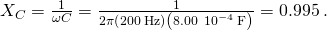2. From (Figure), the inductive reactance is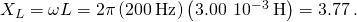3. Substituting the values of R,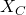, and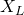into (Figure), we obtain for the impedance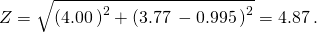4. The current amplitude is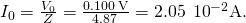5. From (Figure), the phase difference between the current and the emf is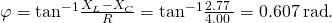Significance The phase angle is positive because the reactance of the inductor is larger than the reactance of the capacitor.

Check Your Understanding Find the voltages across the resistor, the capacitor, and the inductor in the circuit of (Figure) using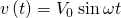as the output of the ac generator.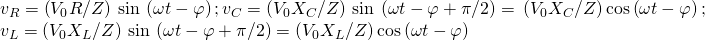### Summary

• An RLC series circuit is a resistor, capacitor, and inductor series combination across an ac source.
• The same current flows through each element of an RLC series circuit at all points in time.
• The counterpart of resistance in a dc circuit is impedance, which measures the combined effect of resistors, capacitors, and inductors. The maximum current is defined by the ac version of Ohm’s law.
• Impedance has units of ohms and is found using the resistance, the capacitive reactance, and the inductive reactance.

### Conceptual Questions

In an RLC series circuit, can the voltage measured across the capacitor be greater than the voltage of the source? Answer the same question for the voltage across the inductor.

yes for both

### Problems

What is the impedance of a series combination of a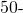resistor, a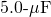capacitor, and a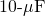capacitor at a frequency of 2.0 kHz?

A resistor and capacitor are connected in series across an ac generator. The emf of the generator is given by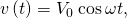where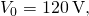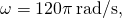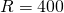, and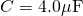. (a) What is the impedance of the circuit? (b) What is the amplitude of the current through the resistor? (c) Write an expression for the current through the resistor. (d) Write expressions representing the voltages across the resistor and across the capacitor.

a.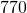; b. 0.16 A; c.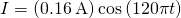; d.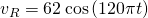;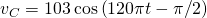A resistor and inductor are connected in series across an ac generator. The emf of the generator is given bywhere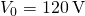and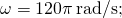also,and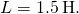(a) What is the impedance of the circuit? (b) What is the amplitude of the current through the resistor? (c) Write an expression for the current through the resistor. (d) Write expressions representing the voltages across the resistor and across the inductor.

In an RLC series circuit, the voltage amplitude and frequency of the source are 100 V and 500 Hz, respectively, an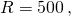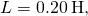and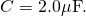(a) What is the impedance of the circuit? (b) What is the amplitude of the current from the source? (c) If the emf of the source is given by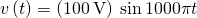, how does the current vary with time? (d) Repeat the calculations with C changed to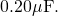a.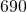; b. 0.15 A; c.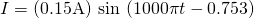; d.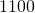, 0.092 A,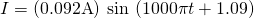An RLC series circuit with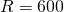,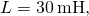and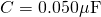is driven by an ac source whose frequency and voltage amplitude are 500 Hz and 50 V, respectively. (a) What is the impedance of the circuit? (b) What is the amplitude of the current in the circuit? (c) What is the phase angle between the emf of the source and the current?

For the circuit shown below, what are (a) the total impedance and (b) the phase angle between the current and the emf? (c) Write an expression for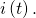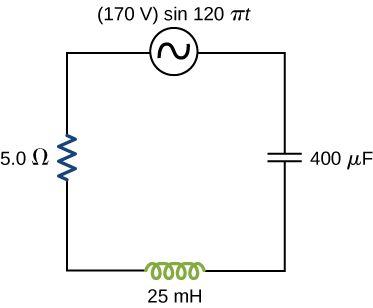a.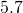; b.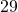; c.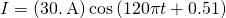### Glossary

impedance
ac analog to resistance in a dc circuit, which measures the combined effect of resistance, capacitive reactance, and inductive reactance
phase angle
amount by which the voltage and current are out of phase with each other in a circuit